# python tutorial - Python String | Python String Methods | Part 1 (find, rfind, startwith, endwith, islower, isupper, lower, upper, swapcase & title) - learn python - python programming

1. find(“string”, beg, end) :- This function is used to find the position of the substring within a string.It takes 3 arguments, substring , starting index( by default 0) and ending index( by default string length).

• It returns “-1 ” if string is not found in given range.
• It returns first occurrence of string if found.

2. rfind(“string”, beg, end) :- This function has the similar working as find(), but it returns the position of the last occurrence of string.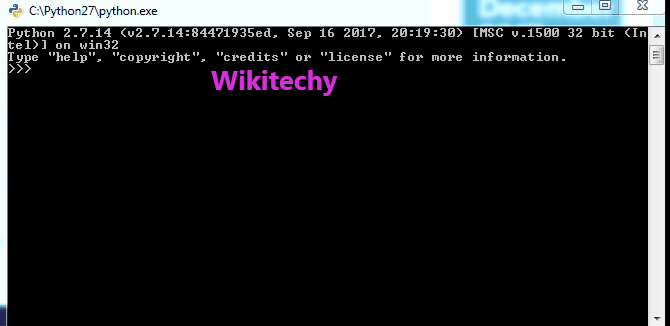Learn Python - Python tutorial - python find - Python examples - Python programs

python - Sample - python code :

python tutorial - Output :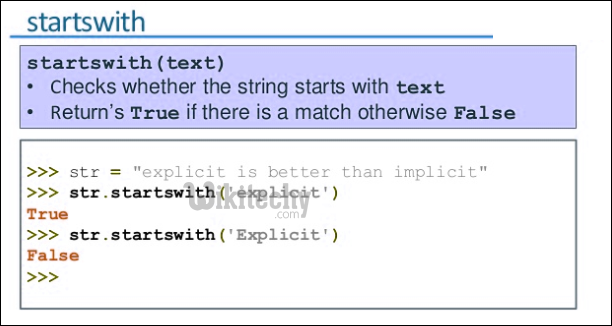3. startswith(“string”, beg, end) :- The purpose of this function is to return true if the function begins with mentioned string(prefix) else return false.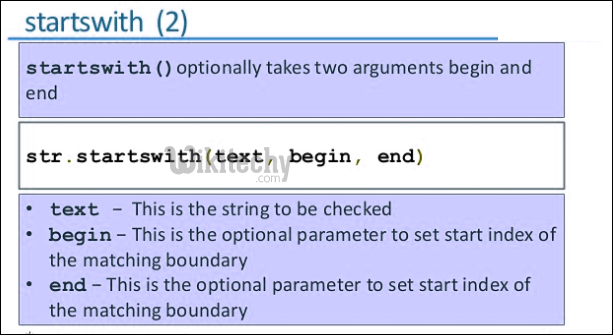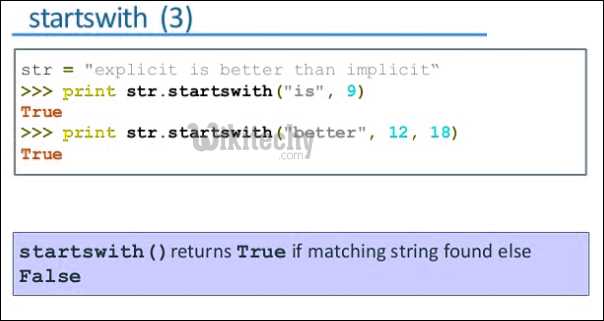4. endswith(“string”, beg, end) :- The purpose of this function is to return true if the function ends with mentioned string(suffix) else return false.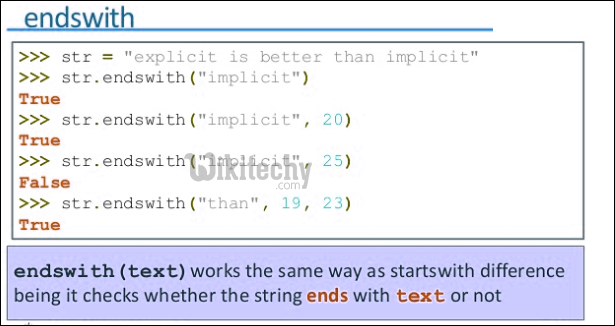python - Sample - python code :

python tutorial - Output :

5. islower(“string”) :- This function returns true if all the letters in the string are

6. isupper(“string”) :- This function returns true if all the letters in the string are upper cased, otherwise false.

python - Sample - python code :

python tutorial - Output :

7. lower() :- This function returns the new string with all the letters converted into its lower case. lower cased, otherwise false.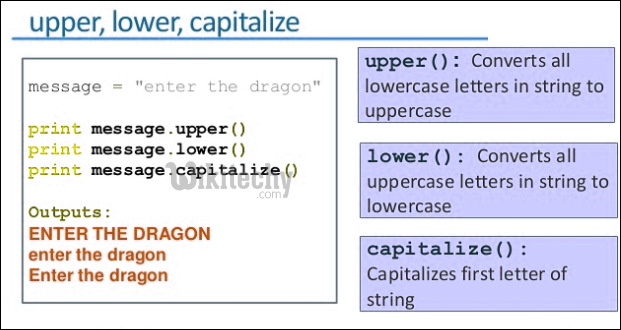8. upper() :- This function returns the new string with all the letters converted into its upper case.

9. swapcase() :- This function is used to swap the cases of string i.e upper case is converted to lower case and vice versa.

10. title() :- This function converts the string to its title case i.e the first letter of every word of string is upper cased and else all are lower cased.

python - Sample - python code :

python tutorial - Output :